# Question 12) Solve the boundary value problem using a program/script that applied the shooting me...

Please provide the program in Matlab.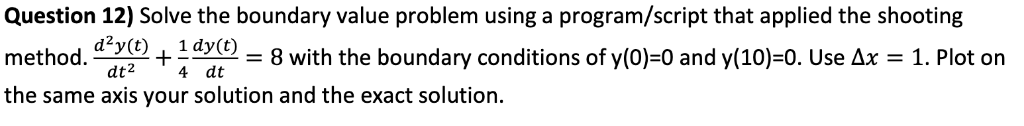Question 12) Solve the boundary value problem using a program/script that applied the shooting method. (t) + y()-8 with the boundary conditions of y(0)-0 and y(10-0. Use ΔΧ-1. Plot on the same axis your solution and the exact solution dt2 t 4 4 dt

syms y(t)

ode = diff(y,x,2)+1/4 * diff(y,x)-8 == 0;

cond1 = y(0) ==0;

cond2 = y(10) ==0;

conds = [cond1 cond2];

ysol(t) = dsolv(ode,conds);

ysol = simplify(ysol)

##### Add Answer of: Question 12) Solve the boundary value problem using a program/script that applied the shooting me...
Similar Homework Help Questions
• ### Set up and solve a boundary value problem using the shooting method using Matlab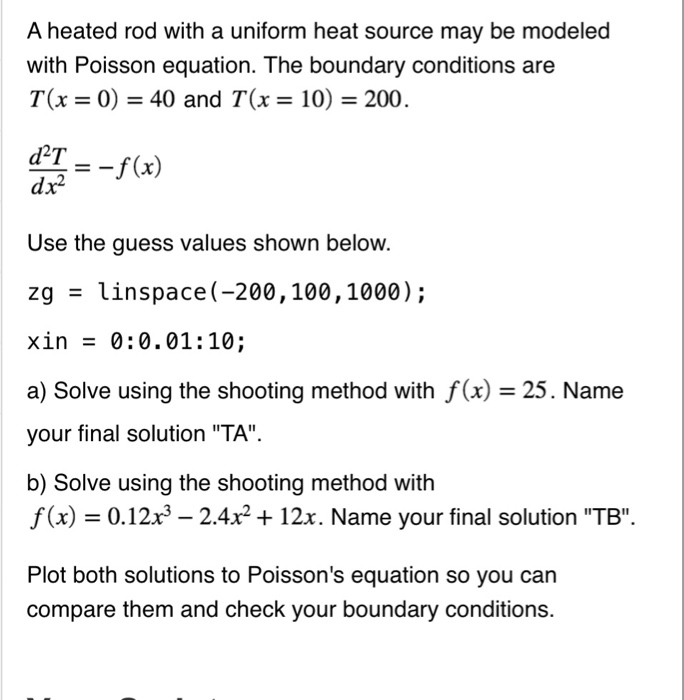Set up and solve a boundary value problem using the shooting method using Matlab A heated rod with a uniform heat source may be modeled with Poisson equation. The boundary conditions are T(x = 0) = 40 and T(x = 10) = 200 dTf(x) Use the guess values shown below. zg linspace (-200,100,1000); xin-0:0.01:10 a) Solve using the shooting method with f(x) = 25 . Name your final solution "TA" b) Solve using the shooting method with f(x)-0.12x3-2.4x2 + 12x....

• ### Problem 2: Use the shooting method to solve d T _-10-7 (T +273)' + 4(150-7): 0 With the boundary ...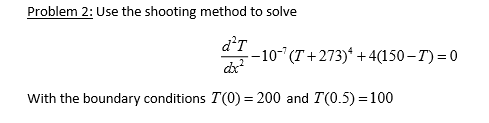solution with matlab only please Problem 2: Use the shooting method to solve d T _-10-7 (T +273)' + 4(150-7): 0 With the boundary conditions T(O)- 200 and T(0.5)-100 Problem 2: Use the shooting method to solve d T _-10-7 (T +273)' + 4(150-7): 0 With the boundary conditions T(O)- 200 and T(0.5)-100

• ### Problem 3. Given the initial conditions, y(0) from t- 0 to 4: and y (0 0, solve the following initial-value problem...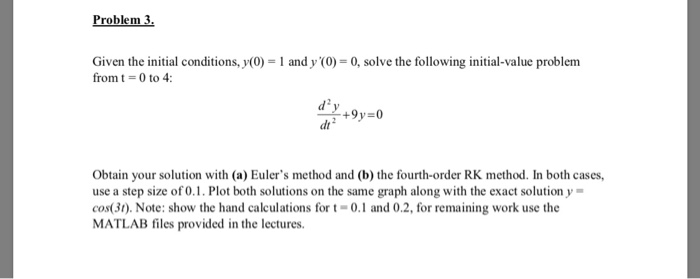Problem 3. Given the initial conditions, y(0) from t- 0 to 4: and y (0 0, solve the following initial-value problem d2 dt Obtain your solution with (a) Euler's method and (b) the fourth-order RK method. In both cases, use a step size of 0.1. Plot both solutions on the same graph along with the exact solution y- cos(3t). Note: show the hand calculations for t-0.1 and 0.2, for remaining work use the MATLAB files provided in the lectures Problem...

• ### answer in matlab code Employ the bvp4c command to find the approximate solution of the boundary value problem governed by the second-order nonhomogeneous differential equation, 9. with the bound...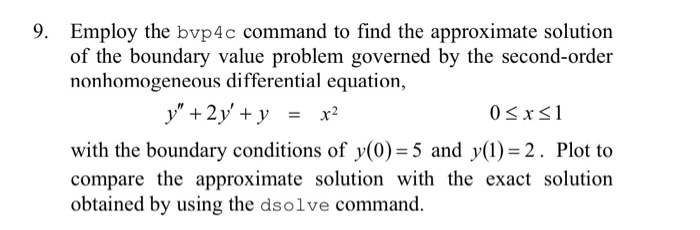answer in matlab code Employ the bvp4c command to find the approximate solution of the boundary value problem governed by the second-order nonhomogeneous differential equation, 9. with the boundary conditions of y(0) 5 and y(1)-2. Plot to compare the approximate solution with the exact solution obtained by using the dsolve command. Employ the bvp4c command to find the approximate solution of the boundary value problem governed by the second-order nonhomogeneous differential equation, 9. with the boundary conditions of y(0) 5...

• ### 03. Consider the boundary value problem 0 Sts1 y(0) & y(1)-1 where k > 0 is a given real paramete...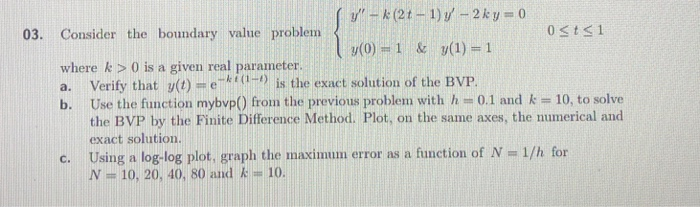03. Consider the boundary value problem 0 Sts1 y(0) & y(1)-1 where k > 0 is a given real parameter a. Verify that y(t) = e-kt (14) is the exact solution of the BVP. b. Use the function mybvp() from the previous problem with h -0.1 and k -10, to solve the BVP by the Finite Difference Method. Plot, on the same axes, the numerical and exact solution. c. Using a log-log plot, graph the maximum error as a function...

• ### NOTE: h=(b - a) / N Consider the differential equation y" y' +2y + cos(), for...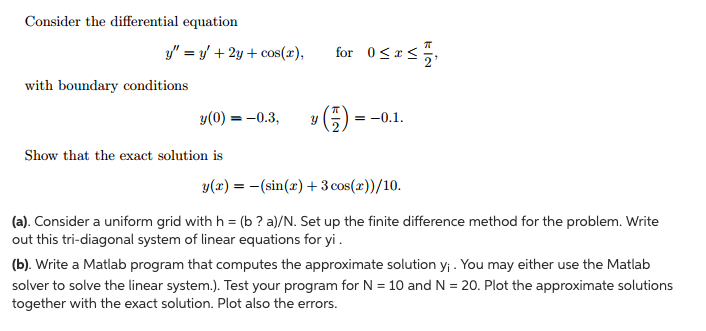NOTE: h=(b - a) / N Consider the differential equation y" y' +2y + cos(), for 0 x , with boundary conditions (0) 0.3, Show that the exact solution is (x)(sin3 cos())/10. (a). Consider a uniform grid with h (b? a)/N. Set up the finite difference method for the problem. Write out this tri-diagonal system of linear equations for yi, (b). Write a Matlab program that computes the approximate solution yi. You may either use the Matlab solver to solve...

• ### I need to solve this using Matlab please type comments in the script so I understand thank you. Create a table (similar to what we do in class) with all the parameters that you have to calculate fo...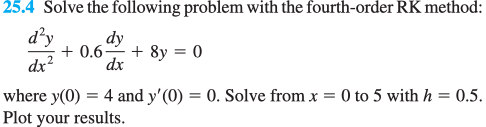I need to solve this using Matlab please type comments in the script so I understand thank you. Create a table (similar to what we do in class) with all the parameters that you have to calculate for every step in the solution. Include y and dy/dx in the same plot with points from your table joined by straight lines (and clearly indicate which line correspond to what). You may use the MATLAB function you created above. Solve the following...

• ### MATLAB (2 points) Challenge. Create a SCRIPT file called thirdOrderDE.m 5) Blasius showed in 1908 that the solution to the incompressible flow field in a laminar boundary layer on a flat plate is...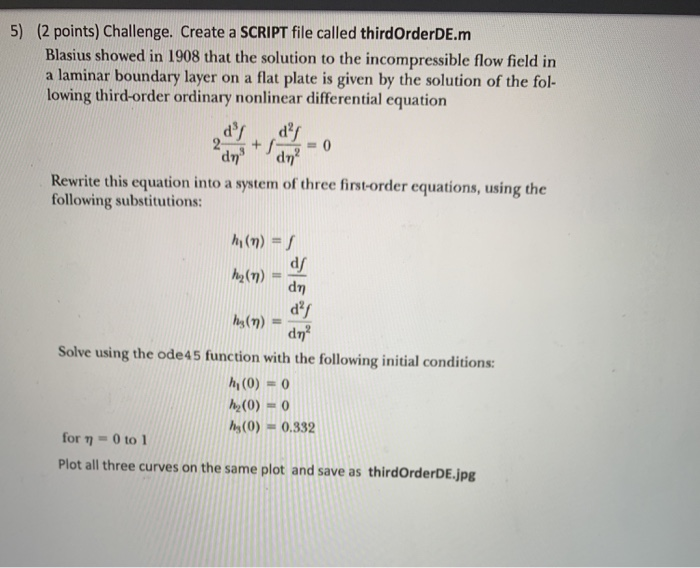MATLAB (2 points) Challenge. Create a SCRIPT file called thirdOrderDE.m 5) Blasius showed in 1908 that the solution to the incompressible flow field in a laminar boundary layer on a flat plate is given by the solution of the fol- lowing third-order ordinary nonlinear differential equation Rewrite this equation into a system of three first-order equations, using the following substitutions: h,(m) = f d2 Solve using the ode45 function with the following initial conditions: hi (0) = 0 hs(0) =...

• ### 3. (a) Solve the boundary value problem on the wedge u(r, 0) = 0 0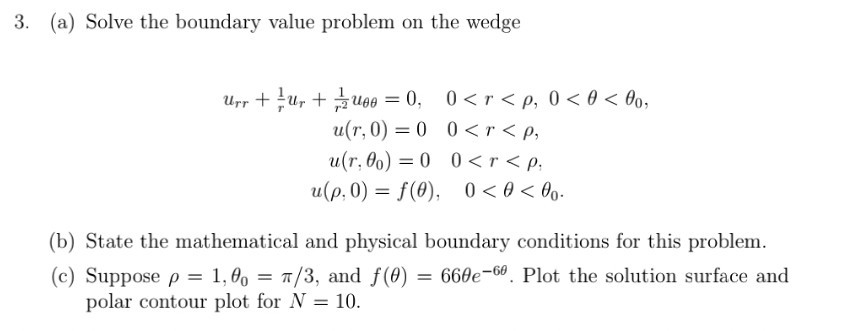3. (a) Solve the boundary value problem on the wedge u(r, 0) = 0 0<r<p, a(r, g) = 0 0<r<p, u(p, 0)-/(0), 0 < θ < θο. (b) State the mathematical and physical boundary conditions for this problem. (c) Suppose ρ-1.00-π/3, and f(9)-66ere. Plot the solution surface and polar contour plot for N -10 3. (a) Solve the boundary value problem on the wedge u(r, 0) = 0 0

• ### please use matlab to solve Problem # 3 P-3 Flow between two paralle plates is described by the following equation dith boundary conditons given as u,-0 & u,-o Calculate the velocity profile u...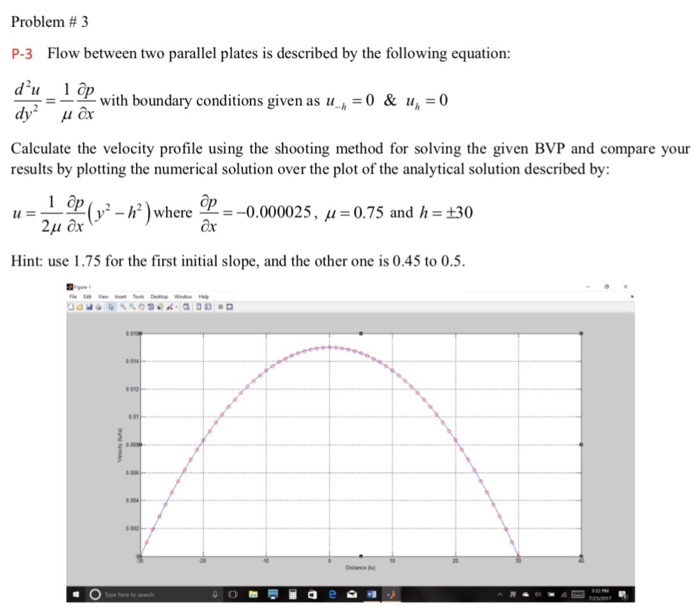please use matlab to solve Problem # 3 P-3 Flow between two paralle plates is described by the following equation dith boundary conditons given as u,-0 & u,-o Calculate the velocity profile using the shooting method for solving the given BVP and compare your results by plotting the numerical solution over the plot of the analytical solution described by: (y-F )where ğr--0000025.H-О75 and h,30 Hint: use 1.75 for the first initial slope, and the other one is 0.45 to 0.5....

Need Online Homework Help?